IMAGINARY NUMBERS
Real numbers include both rational numbers, such as 42 and −23/129, and irrational numbers, such as pi and the square root of two. A real number can be given by an infinite decimal representation, such as 2.4871773339..., where the digits continue in some way. Real numbers may be thought of as points on an infinitely long number line.

Imaginary numbers are in the form "bi" where "b" is a non-zero, real number and "i" is defined by i² = -1,  called the "imaginary unit". An Imaginary number bi can be added to a real number a, to form a "complex number" of the form (a + bi), where a and b are called respectively, the "real part" and the "imaginary part" of the complex number. Imaginary numbers can therefore be thought of as complex numbers where the real part is zero. The square of an imaginary number is a negative real number.

The complex conjugate of a complex number has the reverse sign of the original imaginary part. The complex conjugate of (a + bi) is (a - bi).

Real and imaginary parts are additive: (a + bi) + (c +di) = (a + c) + (b + d)i, and are multiplicative:
(a + bi) * (c + di) = ac + bci +adi + bdi2 = (ac - bd) + (bc + ad)i.

Division is accomplished by multiplying the numerator and denominator by the complex conjugate of the denominator: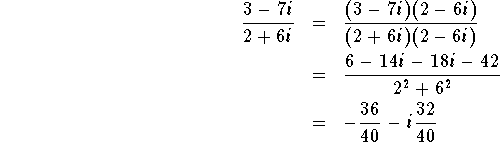BASIC ALGEBRA
Elementary algebra is one of the main branches of pure mathematics and introduces the concept of variables representing numbers. Statements based on these variables are manipulated using the rules of operations that apply to numbers, such as addition. This can be done for a variety of reasons, including equation solving. While the word algebra comes from the Arabic languageand much of its methods from Arabic/Islamic mathematics, its roots can be traced to earlier traditions, most notably ancient Indian mathematics.A polynomial is an expression that is constructed from one or more variables and constants, using only the operations of addition, subtraction, multiplication, and exponentiation. For example, x2 + 2x − 3 is a polynomial in the single variable x.

Factoring of polynomials is the process of expressing a given polynomial as a product of other polynomials. The example polynomial above can be factored as (x − 1)(x + 3).

A quadratic equation is one in which a term is raised to the power of 2 and other terms are raised to a lower power or a are constants: x2 + 2x − 3 =0 is a quadradic equation.

A root, or zero,  of a polynomial in a single variable is the value that of x that forces the polynomial to produce a result equal to zero. In the example above, x = 1 and x = -3 are roots or zeros of the polynomial x2 + 2x − 3 = (x − 1)(x + 3) = 0. All real polynomials of odd degree have a real number as a root. Many real polynomials of even degree do not have a real root.

A polynomial of degree n will have n roots. Roots are not always obvious. The roots of a quadratic equation are found with:Roots of higher order equations are solved by graphing or iterative "solver" software.

Linear simultaneous equations - see Cramer's Rule.
Statistical analysis methods - see StatisticsBASIC GEOMETRY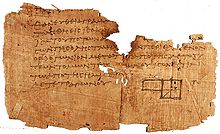Plane geometry is the study of angles and triangles, perimeter, area and volume. It differs from algebra in that it develops a logical structure where mathematical relationships are proved and applied. Euclid (c. 300 BCE) introduced certain axioms, or postulates, expressing primary or self-evident properties of points, lines, and planes. He proceeded to rigorously deduce other properties by mathematical reasoning. Some of these are listed below.

A point shows position.

A line is infinite and straight and is a set of continuous points that extend indefinitely in either of its direction. A line segment is part of the straight line between two points.

Parallel lines do not cross.

A ray is the part of the line which consists of a given point and the set of all points on one side of that point.

A circle is a set of points equidistant from another point, known as the center of the circle.

An angle can be defined as two rays or two line segments having a common end point. The endpoint becomes known as the vertex. An angle occurs when two rays meet or unite at the same endpoint.

A plane is a flat surface containing three points that are not all in a straight line.

The intersection of two plones is a line.

###2-D AREAS Square: A = a2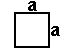Rectangle: A = a * b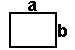Parallelogram: A = b * hTrapezoid: A = h * (b1 + b2) / 2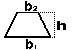Circle: A = pi *  r 2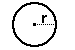Ellipse: A = pi * r1 * r2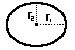Triangle: A = 0.5 * b * h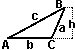Equilateral triangle A = 0.25 *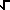(3) * a2

Triangle given SAS: A = 0.5 * a * b * sin C

Triangle given a,b,c: A =[s * (s - a) * (s - b) * (s - c)] when s = (a + b + c) / 2

Regular polygon: A = 0.5 * n * sin(360°/n) * S2
where:  n = # of sides and S = length from center to a corner

###3-D VOLUMES

Cube: V = a3Rectangular prism: V = a * b * c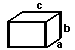Irregular prism: V = b * hCylinder: V  = b * h = pi *  r2 * hPyramid: V = 0.5 * b * hCone: V = (1/3) * b * h = (1/3) * pi * r2 * hSphere: V = (4/3) * pi * r3Ellipsoid: V= (4/3) * pi * r1  * r2 * r3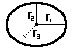###3-D Surface Areas

Cube: S = 6 * a2Prism:
Lateral Area S = perimeter(b) * L
Total Area S = perimeter(b) * L + 2 * bSphere: S = 4 * pi * r2BASIC TRIGONOMETRYTrigonometry is a branch of mathematics that studies triangles on a plane surfaces and deals with relationships between the sides and the angles of triangles and with the trigonometric functions, which describe those relationship.Basic Conversions
PI = 3.141 592...  (approximately 22/7 = 3.1428)

radians = degress x PI / 180
degress = radians x 180 / PI
e = 2.718 282....Trigonometry Functions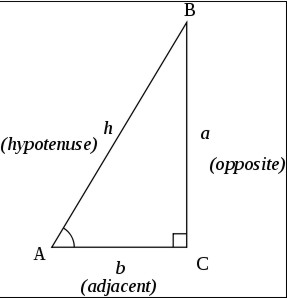The trigonometry functions are defined by a right triangle with angle theta, adjacent side b, oposite side a, and hypotenuse c.

sin(theta) = a / c

csc(theta) = 1 / sin(theta) = c / a

cos(theta) = b / c

sec(theta) = 1 / cos(theta) = c / b

tan(theta) = sin(theta) / cos(theta) = a / b

cot(theta) = 1/ tan(theta) = b / aUSEFUL EQUALITIES
sin^2(x) + cos^2(x) = 1
tan^2(x) + 1 = sec^2(x)
cot^2(x) + 1 = csc^2(x)
sin(xy) = sin x cos ycos x sin y
cos(xy) = cos x cos y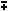sin x sin y

sin(-x) = -sin(x)
csc(-x) = -csc(x)
cos(-x) = cos(x)
sec(-x) = sec(x)
tan(-x) = -tan(x)
cot(-x) = -cot(x)

tan(xy) = (tan xtan y) / (1tan x tan y)
sin(2x) = 2 sin x cos x
cos(2x) = cos^2(x) - sin^2(x) = 2 cos^2(x) - 1 = 1 - 2 sin^2(x)
tan(2x) = 2 tan(x) / (1 - tan^2(x))
sin^2(x) = 1/2 - 1/2 cos(2x)
cos^2(x) = 1/2 + 1/2 cos(2x)
sin x - sin y = 2 sin( (x - y)/2 ) cos( (x + y)/2 )
cos x - cos y = -2 sin( (x-y)/2 ) sin( (x + y)/2 )

Table of Common Angles
Angle 0 30 45 60 90
sin^2(a) 0/4 1/4 2/4 3/4 4/4
cos^2(a) 4/4 3/4 2/4 1/4 0/4
tan^2(a) 0/4 1/3 2/2 3/1 4/0

Given Triangle abc, with angles A,B,C; a is opposite to A, b oppositite B, c opposite C:
Then: a / sin(A) = b / sin(B) = c / sin(C)
c^2 = a^2 + b^2 - 2ab cos(C) Law of Sines
b^2 = a^2 + c^2 - 2ac cos(B)
a^2 = b^2 + c^2 - 2bc cos(A) Law of Cosines

(a - b)/(a + b) = tan 1/2(A-B) / tan 1/2(A+B) (Law of Tangents)

###Hyperbolic Definitions   sinh(x) = ( ex - e-x )/2   csch(x) = 1/sinh(x) = 2/( ex - e-x )   cosh(x) = ( e x + e -x )/2   sech(x) = 1/cosh(x) = 2/( ex + e-x )   tanh(x) = sinh(x)/cosh(x) = ( ex - e-x )/( ex + e-x )   coth(x) = 1/tanh(x) = ( ex + e-x)/( ex - e-x )

cosh2(x) - sinh2(x) = 1
tanh2(x) + sech2(x) = 1
coth2(x) - csch2(x) = 1

###Spherical Trigonometry Spherical trigonometry is a branch of spherical geometry which deals with polygons (especially triangles) on the sphere and the relationships between the sides and the angles. This is of great importance for calculations in astronomy and earth-surface, orbital and space navigation.

On the surface of a sphere, the closest analogue to straight lines are great circles, i.e. circles whose center coincide with the center of the sphere. As with a line segment in a plane, an arc of a great circle (subtending less than 180°) on a sphere is the shortest path lying on the sphere between its two endpoints. An area on the sphere, bounded by arcs of great circles, is called a spherical polygon.

The sides of these polygons are specified not by their lengths, but by the angles at the sphere's center subtended by the endpoints of the sides. Note that this arc angle, measured in radians, when multiplied by the sphere's radius equals the arc length.The sum of the vertex angles of spherical triangles is always larger than the sum of the angles of plane triangles, which is exactly 180°. The amount E by which the sum of the angles exceeds 180° is called spherical excess:
E = A + B + C - pi

where A, B, and C denote the angles.

This surplus determines the surface area of any spherical triangle:
A = R2 * E

where R is the radius of the sphere.